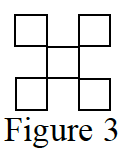Home > CCA > Chapter Ch6 > Lesson 6.2.1 > Problem6-66

6-66.

Figure 3 of a tile pattern is shown at right. If the pattern grows linearly and if Figure 7 has 13 tiles, then find an equation for the pattern. Homework Help ✎Use the information to write points, for example (7, 13). Use the points to write the equation.

y = 2x − 1